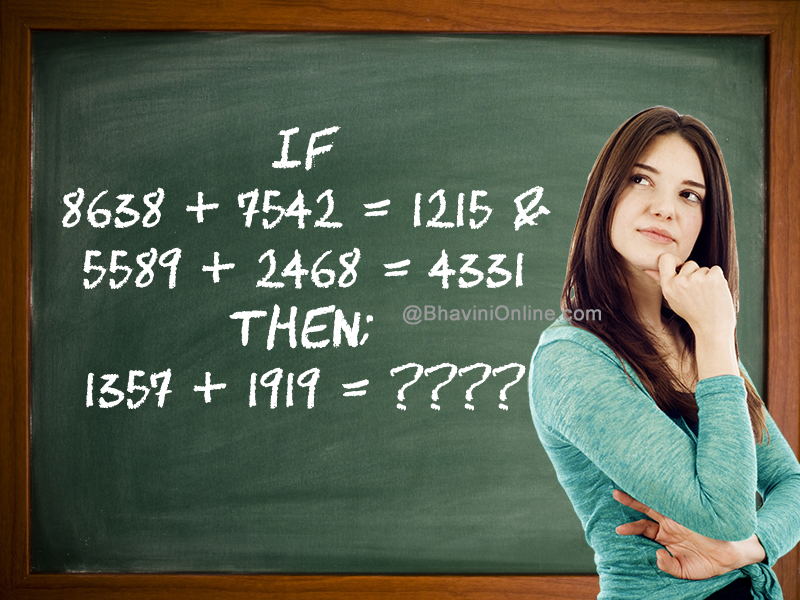# Fun Math Riddle: If 8638 + 7542 = 1215 Then; 1357 + 1919 = ????

Look at the given equations and find the logic behind them.

Once you have cracked the connection between the numbers, find the missing numbers in the last equation.

If

8638 + 7542 = 1215 &

5589 + 2468 = 4331

Then;

1357 + 1919 = ????If you get the correct answer, share it with your friends on WhatsApp, Facebook and other social networking sites.